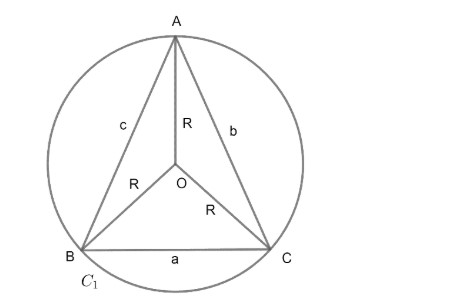Courses
Courses for Kids
Free study material
Offline Centres
MoreLast updated date: 26th Nov 2023
Total views: 381.9k
Views today: 10.81k

# If R is the radius of circumcentre of $\Delta ABC,$ then $R=\dfrac{abc}{4S}$ (A) True (B) FalseVerified
381.9k+ views
Hint: Use area of triangle formula, where two sides of triangle and angle between them is given. Use sine rule related with circumradius to get the given relation.

Here, we have given R as a radius of circumcircle i.e. circumradius and need to prove the relation;
$R=\dfrac{abc}{4S}$……………….(1)
Where (a, b, c) are sides of the triangle as denoted in the diagram.Where O is the centre of the circle C, which is circumscribing the triangle ABC.
R = Circumradius of triangle ABC.
We can write sine rule in $\Delta ABC$ involving circumradius R as;
$\dfrac{\sin A}{a}=\dfrac{\sin B}{b}=\dfrac{\sin C}{c}=\dfrac{1}{2R}..............\left( 2 \right)$
As we have a formula of area with involvement of two sides and angle between them.
Let the area be represented by S.
$Area=S=\dfrac{1}{2}bc\sin A=\dfrac{1}{2}ab\sin C=\dfrac{1}{2}ac\sin B........\left( 3 \right)$
Now, from equation (2) and (3), we can write an equation with respect to one angle as
$\dfrac{\sin A}{a}=\dfrac{1}{2R}\text{ and }S=\dfrac{1}{2}bc\sin A$
Substituting value of sin A from the relation $\dfrac{\sin A}{a}=\dfrac{1}{2R}\text{ to }S=\dfrac{1}{2}bc\sin A$, we get;
As $\sin A=\dfrac{a}{2R}$ from the first relation, now putting value of sin A in $S=\dfrac{1}{2}bc\sin A$, we get
\begin{align} & S=\dfrac{1}{2}bc\dfrac{a}{2R} \\ & S=\dfrac{abc}{4R} \\ \end{align}
Transferring R to other side, we get;
$R=\dfrac{abc}{4S}$
Hence, the relation given in the problem is true.
Note: One can go wrong with the formula of area of the triangle. One can apply heron’s formula for proving i.e.
\begin{align} & S=\sqrt{s\left( s-a \right)\left( s-b \right)\left( s-c \right)} \\ & s=\dfrac{a+b+c}{2} \\ \end{align}
Which will make the solution very complex.
One can go wrong while writing sine rule as;
$\dfrac{\sin A}{a}=\dfrac{\sin B}{b}=\dfrac{\sin C}{c}=\dfrac{2R}{1}$ which is wrong.
Correct equation of sine rule will be,
$\dfrac{\sin A}{a}=\dfrac{\sin B}{b}=\dfrac{\sin C}{c}=\dfrac{1}{2R}$.